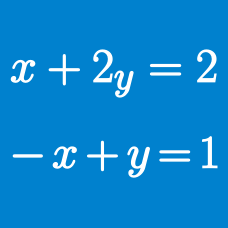Algebra

# System of Equations - Factorization

If complex numbers $x$ and $y$ satisfy the simultaneous equations \begin{aligned} x^2+y^2 &= 4 \\ 2x^2-3y^2 &= -67, \end{aligned} what is the value of $(y+x)(y-x)$?

Let $a$ and $b$ be the values of non-zero real numbers $x$ and $y,$ respectively, that satisfy $3x^2+18y-12x=-9, x^2+9y-7x=-6.$ What is the value of $a^2+b^2?$

Let $x=A$ and $y=B$ be the solutions of the simultaneous equations \begin{aligned} \frac{3}{2x-1}+\frac{59}{y+1} &= 2 \\ \frac{3}{2x-1}-\frac{59}{y+1} &= 1. \end{aligned} What is the value of $\frac{B}{A}$?

If $x=a \; (< 0)$ and $y=b$ are solutions of the simultaneous equations $2x^2-2y^2+3x-56y=44,\, x^2-y^2+x-29y=22,$ what is the value of $b-a$?

Let $x=\alpha$, $y=\beta$ and $z=\gamma$ be the solutions of the simultaneous equations \begin{aligned} x+y-19xy &= 0 \\ y+z-12yz &= 0 \\ z+x-21zx &= 0, \end{aligned} where $xyz \neq 0$. If the value of $\alpha+\beta+\gamma$ can be expressed as $\frac{a}{b}$, where $a$ and $b$ are coprime positive integers, what is $a+b$?

×